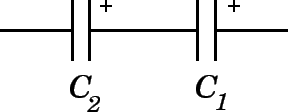# Question dd1e3

Jan 4, 2015

For capacitors in parallel:The total capacitance C_(TOT# is given by:

${C}_{T O T} = {C}_{1} + {C}_{2}$

Since ${C}_{T O T} = \frac{Q}{V}$ the total can be found from $Q = {C}_{T O T} V$ where V is the voltage drop across the capacitors.

For capacitors in series:$\frac{1}{C} _ \left(T O T\right) = \frac{1}{C} _ 1 + \frac{1}{C} _ 2$

So $Q = {C}_{T O T} V$